Get ready for a maths double-whammy! Add up the equations and compare them using greater than or less than symbols.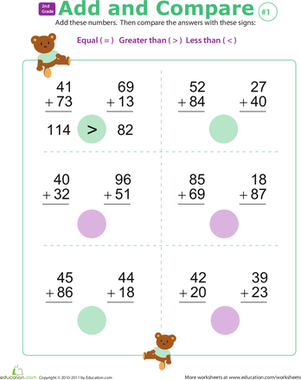### Greater Than Less Than Symbols

Kids solve two-digit addition problems, then compare the answers using the greater than, less than, and equal to symbols in this year 3 maths worksheet.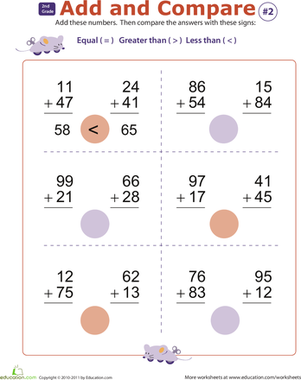In this year 3 addition worksheet, your child will learn how to compare numbers using the greater than, less than, and equal to mathematical symbols.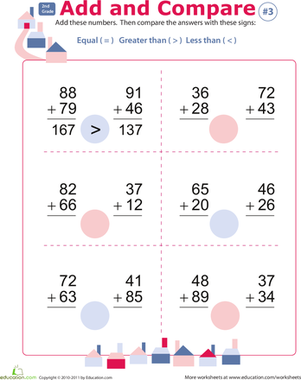Children learn how to compare numbers using the greater than and less than symbols in this cheerful year 3 addition worksheet.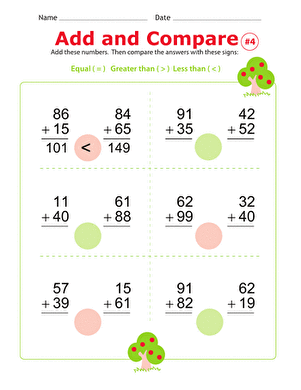With a lesson on comparing numbers using the greater than and less than symbols, this year 3 maths worksheet adds an extra dimension to addition practise.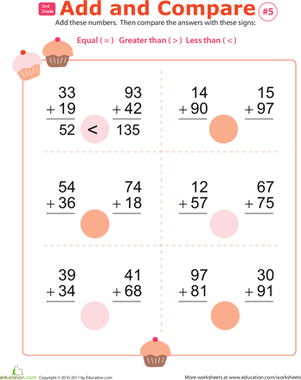In this worksheet, your child will solve two-digit addition problems, then choose the symbol that correctly completes each inequality.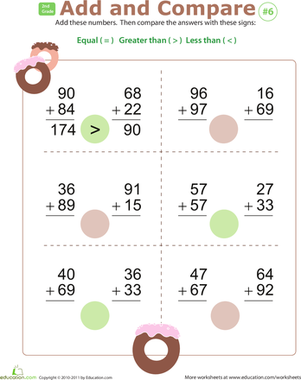This year 3 addition worksheet offers a gentle introduction to greater than and less than statements, also known as inequalities.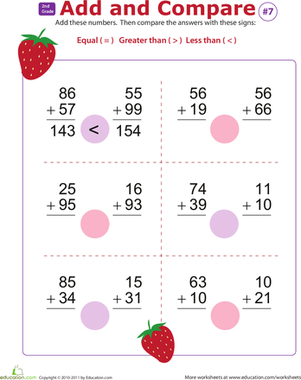Introduce your second grader to inequalities, also called greater than and less than statements, with this cheery strawberry-themed addition worksheet.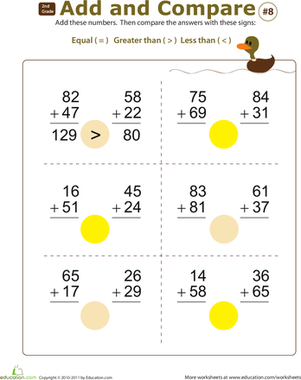Combining advanced two-digit addition with inequality statements, this year 3 maths worksheet really packs a punch.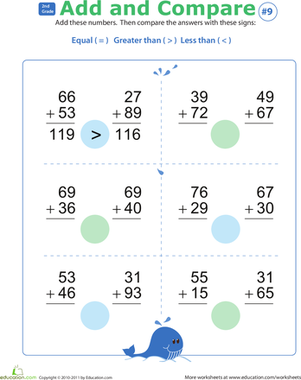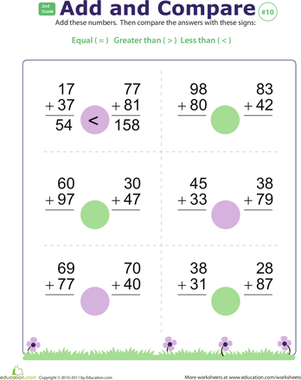Create new collection

0

### New Collection>

0Items

What could we do to improve Education.com?